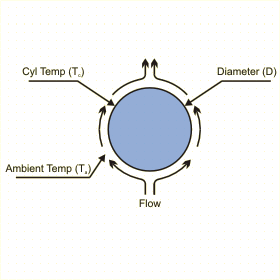This page calculates the heat transfer coefficient, heat convected and heat radiated from an isothermal horizontal cylinder assuming natural convection. The convection calculation is based on Rayleigh number and is valid for Rayleigh numbers between 10-5 and 1012. The ends of the cylinder are assumed to be adiabatic. Define the channel size, cylinder temperature and ambient air fluid temperature. You also need to define the fluid properties. This page will calculate a heat transfer coefficient based on the flow conditions as well as the heat convected from the cylinder. To account for convection only, make the emissivity equal to 0. Similarly, to account for radiation only, make the tehrmal conductivity = 0.Do you want to know more ?Cylinder and Flow Conditions

Fluid Properties at Film Temperature (Default is for Air at 20C)

 Description Symbol Value Units Film Temperature = (Tc+Ta)/2 Tf oC Specific Heat Cp J/kg-oC Coefficient of Thermal Expansion B 1/K Dynamic Viscosity µ kg/m-s Density at reference temperature of 20 C rhoref kg/m3 Density calculated at film temperature Tf rho kg/m3 Thermal Conductivity k W/m-oC Emissivity e None

Cylinder Dimensions

 Description Symbol Value Units Length L Meters Diameter D Meters Cylinder Temperature Tc oC Ambient Temperature Ta oC

Results for Cylinder

 Description Symbol Value Units Prandlt Number = Cp*µ / k Pr None Area = PI * D A m2 Rayleigh Number = gB rho2Cp(Tc-Ta)D3 / kµ Ra None Nusselt = {0.60+(0.387RaD1/6) / [1+(0.559/Pr)9/16](8/27)}2 Nu None Average Heat Transfer Coefficient = Nu*k/HD h W/m2-C Convective Heat Transfer = hA(Tc-Ta) qconv W Radiative Heat Transfer qrad W Total Heat Transfer q W
 Copyright and Disclaimer Noticepowered by MAYA HTT